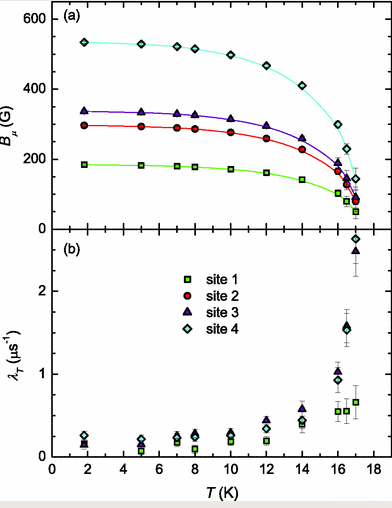# CuSe2O5¶

This example reproduces the results of PhysRevB 87 104413 and shows how to use mago_add

In :

import numpy as np
from muesr.engines.clfc import locfield
from muesr.core import Sample
from muesr.utilities import show_structure

         Spg Library not loaded


The following method generates a rotation matrix

In :

def rotation_matrix(axis, theta):
"""
Return the rotation matrix associated with counterclockwise rotation about
the given axis by theta radians.
"""
axis = np.asarray(axis)
theta = np.asarray(theta)
naxis = axis/np.linalg.norm(axis)
a = np.cos(theta/2.0)
b, c, d = -naxis*np.sin(theta/2.0)
aa, bb, cc, dd = a*a, b*b, c*c, d*d
bc, ad, ac, ab, bd, cd = b*c, a*d, a*c, a*b, b*d, c*d
[2*(bd+ac), 2*(cd-ab), aa+dd-bb-cc]])


Define the CuSe2O5 object, load the structure from the cif file and visualize it for a first check. Remember to close XCrysDen or VESTA.

In :

s = Sample()
show_structure(s) # show_structure(s,visualizationTool='V') if you want VESTA

Out:

True


Cu atoms are 1,2,3,4. According to Phys Rev B 87 104413 Cu moments in Bohr magnetons are: | 0.13 0.50 0.00 | | 0.13 -0.50 0.00 | | -0.13 -0.50 0.00 | | -0.13 0.50 0.00 | (Copy-Paste in mago_add below)

In :

kvalue = np.array([0.,0.,0.])


if kvalue is the propagation vector (0,0,0 amd 1,0,0 are the same!). Thus the magnetic structure can be added to s with mago_add. A prompt will appear (also under Jupyter) asking first for the name of the magnetic ion [Cu] and then for the Fourier components

In :

mago_add(s,coordinates='b-l', kvalue=kvalue) # using Bohr magnetons and Cartesian coordinates

         Fourier components in Bohr magnetons and lattice coordinates.
Which atom? (enter for all): Cu
Lattice vectors:
a   12.254000000000000    0.000000000000000    0.000000000000000
b    0.000000000000000    4.858000000000000    0.000000000000000
c   -2.813659756482887    0.000000000000001    7.446134485405744
Atomic positions (fractional):
1 Cu  0.00000000000000  0.00000000000000  0.00000000000000  63.546
2 Cu  0.00000000000000  0.00000000000000  0.50000000000000  63.546
3 Cu  0.50000000000000  0.50000000000000  0.00000000000000  63.546
4 Cu  0.50000000000000  0.50000000000000  0.50000000000000  63.546
FC for atom 1 Cu (3 real, [3 imag]):  0.13 0.50 0.00
FC for atom 2 Cu (3 real, [3 imag]):  0.13 -0.50 0.00
FC for atom 3 Cu (3 real, [3 imag]):  -0.13 -0.50 0.00
FC for atom 4 Cu (3 real, [3 imag]):  -0.13 0.50 0.00

Out:

True


Let’’s add four tentative muon sites and compute the result in the a*bc coordinate system by applying a rotation of 110.7-90 = -20.7 degrees (read the paper)

In :

s.add_muon([0.19,0.01,0.23])

show_structure(s,visualizationTool='V')   # check magnetic structure

Out:

True

In :

results = locfield(s,'s',[50,50,50],100)
print("In the A*bc coordinate system the fields are:")
for i, f in enumerate(results):
# rotate in a*bc coordinate system
Bv = np.dot(f.D,rotation_matrix([0,1,0],-np.pi*(20.7/180)))
B = np.sqrt(np.dot(f.D,f.D))
print("Site ",i,", B = ", Bv, " T, |B| = ",B," T")

In the A*bc coordinate system the fields are:
Site  0 , B =  [ 0.00572451 -0.01632982  0.00716516]  T, |B| =  0.018728922602301346  T
Site  1 , B =  [-0.0193075   0.01199599  0.02114259]  T, |B| =  0.031043394671416997  T
Site  2 , B =  [-0.02136884  0.01998901  0.01386566]  T, |B| =  0.03237969132689694  T
Site  3 , B =  [-0.00625175 -0.05112271 -0.01505342]  T, |B| =  0.0536583755500242  T


Compare with figure 6 from PRB 87 104413